Vectors and conics

This free course is available to start right now. Review the full course description and key learning outcomes and create an account and enrol if you want a free statement of participation.

Free course

4.5 Ellipse (0 < e < 1)

An ellipse with eccentricity e (where 0 < e < 1) is the set of points P in the plane whose distances from a fixed point F are e times their distances from a fixed line d. We obtain such an ellipse in standard form if

1. the focus F lies on the x-axis, and has coordinates (ae, 0), where a > 0;

2. the directrix d is the line with equation x = a/e.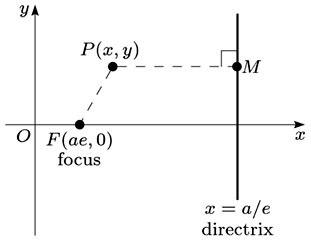Let P (x, y) be an arbitrary point on the ellipse, and let M be the foot of the perpendicular from P to the directrix. Since FP = e × PM, by the definition of the ellipse, it follows that FP2 = e2 × PM2; that is,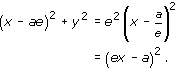Multiplying out the brackets, we obtain

x2 − 2aex + a2e2 + y2 = e2x2 − 2aex + a2,

which simplifies to the equation

x2(1 − e2) + y2 = a2(1 − e2),

that is,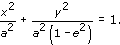Substituting b for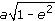, so that b2 = a2(1 − e2), we obtain the standard form of the equation of the ellipse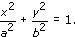This equation is symmetric in x and in y, so that the ellipse also has a second focus F′ at (−ae, 0), and a second directrix d′ with equation x = −a/e.

The ellipse intersects the axes at the points (±a, 0) and (0, ±b). We call the line segment joining the points (±a, 0) the major axis of the ellipse, and the line segment joining the points (0, ±b) the minor axis of the ellipse. Since b < a, the minor axis is shorter than the major axis. The origin is the centre of this ellipse.

Note: Since 0 < e < 1, we have 0 < b < a.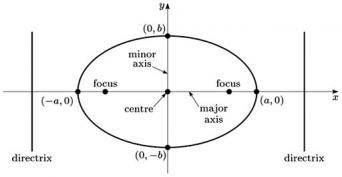Each point with coordinates (a cos t, b sin t) lies on the ellipse, since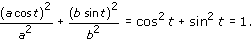Then, just as for the parabola, we can check that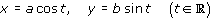gives a parametric representation of the ellipse.

An ellipse with eccentricity e = 0 is a circle. In this case, a = b and the circle x2 + y2 = a2 can be parametrised by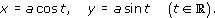We summarise these facts about ellipses (including circles) as follows:

Ellipse in standard form

An ellipse in standard form has equation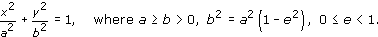It can also be described by the parametric equations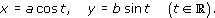If e > 0, it has foci (±ae, 0) and directrices x = ±a/e; its major axis is the line segment joining the points (±a, 0), and its minor axis is the line segment joining the points (0, ±b).

If e = 0, the ellipse is a circle.

Example 56

Let P be a point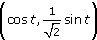, t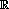, on the ellipse with equation x2 + 2y2 = 1.

• (a) Determine the foci F and F′ of the ellipse.

• (b) Determine the gradients of FP and FP, when these lines are not parallel to the y-axis.

• (c) Find those points P on the ellipse for which FP is perpendicular to FP.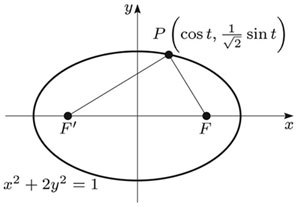• (a) This ellipse is of the form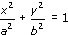with a = 1 and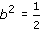, so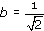If e denotes the eccentricity of the ellipse, so that b2 = a2(1 − e2), then we have

•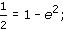• it follows that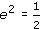, so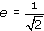.

• In the general case, the foci are (±ae, 0); it follows that here the foci are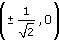.

• (b) Let F and F′ be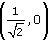and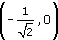, respectively. (It does not matter which way round these are chosen.)

• Then the gradient of FP is

•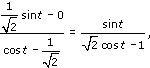• where we know that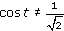, since FP is not parallel to the y-axis.

• Similarly, the gradient of FP is

•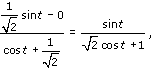• where we know that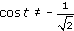, since FP is not parallel to the y-axis.

• (c) When FP is perpendicular to FP, we have

•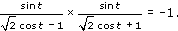• We may rewrite this in the form

•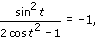• so 2 cos2t − 1 + sin2t = 0.

• Since cos2t + sin2t = 1, it follows that cos2t = 0 and so cost = 0. This occurs only when t = ±/2; that is, at the points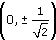.

M208_1